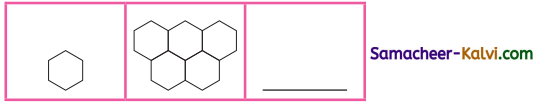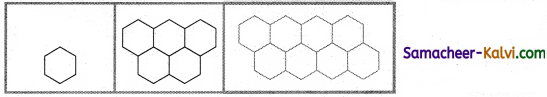## Samacheer Kalvi 3rd Standard Maths Book Solutions Term 3 Chapter 1 Geometry

Question 1.
Draw the diagonals for the given rectangle.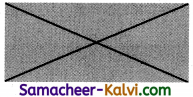Question 2.
How many diagonals will be there in a cuboid?
16Match the properties of 2D shapes by observing their sides and corners (vertices).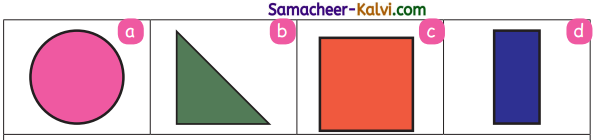Question 1.
Opposite sides are equal.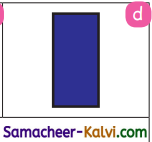Question 2.
There are no sides and corners.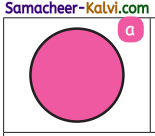Question 3.
Sides may or may not be equal.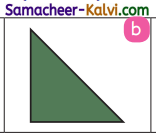Question 4.
All the four sides are equal.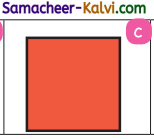Properties of 3D Objects:

Question 1.
Pick out the shapes with (i) curved surfaces (ii) flat surfaces (iii) curved and flat surfaces from the given picture and completed table.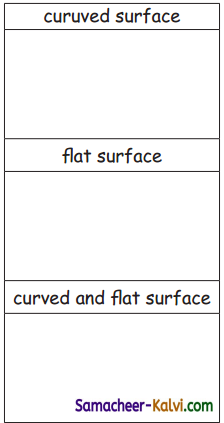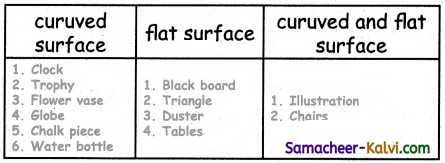Question 2.
Complete the table by counting the sides, corners and diagonals of the given 3D shapes.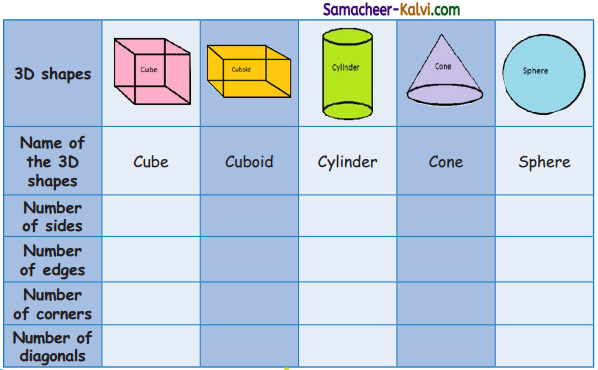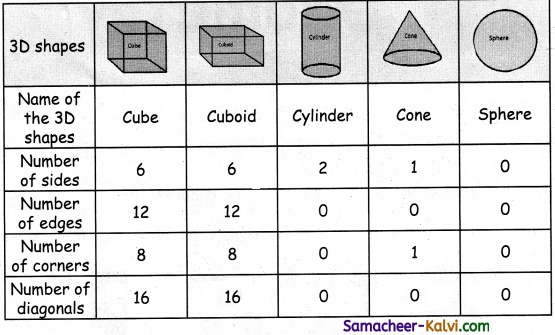Tangrams (Text Book Page No. 7):

Identify the tangram pieces used in the given images by colouring and numbering the pieces as in the reference figure.

Question 1.
A tangram house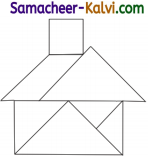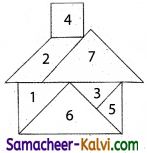Question 2.
A tangram boat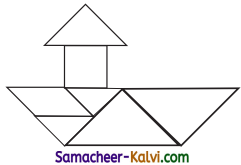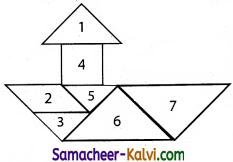Question 3.
A tangram swan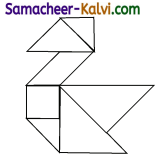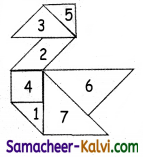Tessellation (Text Book Page No. 8):

Question 1.
Complete the shapes by filling the tiles.

(i)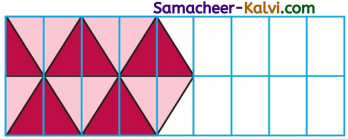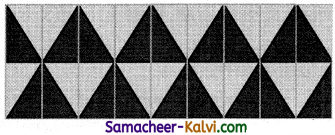(ii)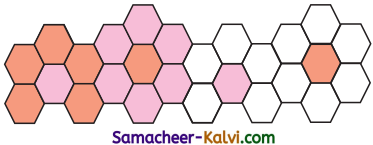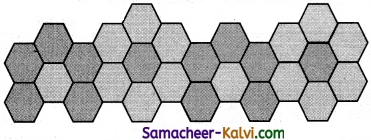Question 2.
Draw one more tile to continue the pattern.

i)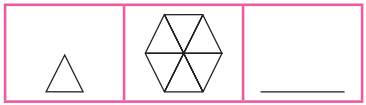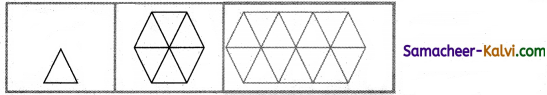ii)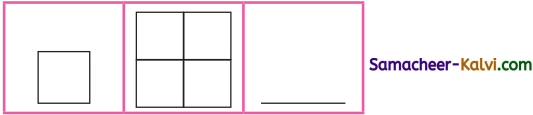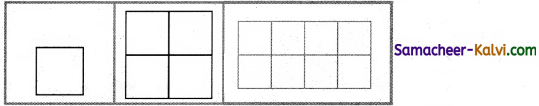iii)